Anúncio

# Discovery of Linear Acyclic Models Using Independent Component Analysis

3 de Dec de 2014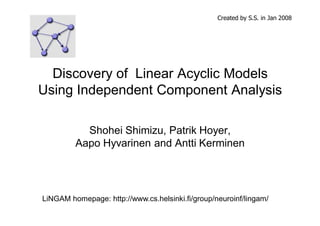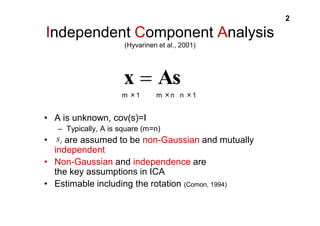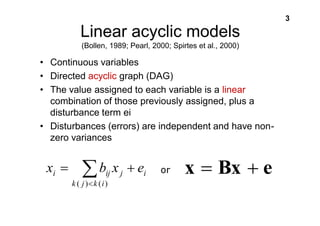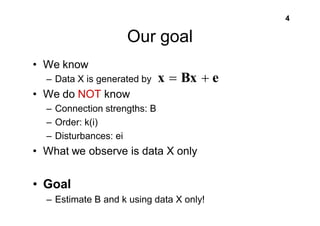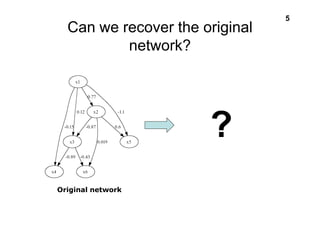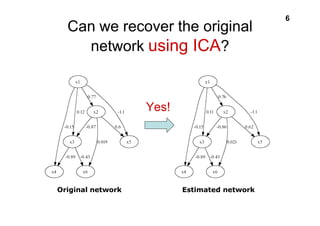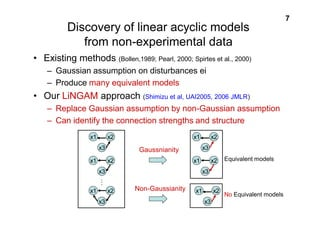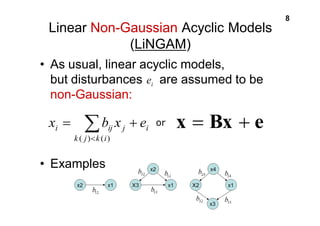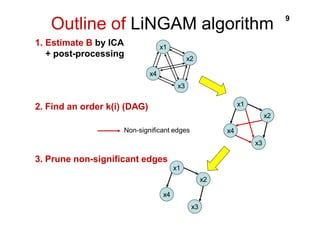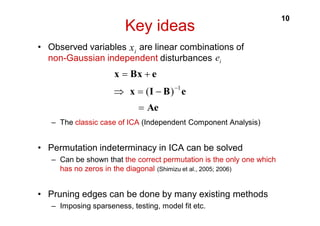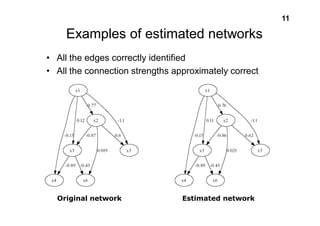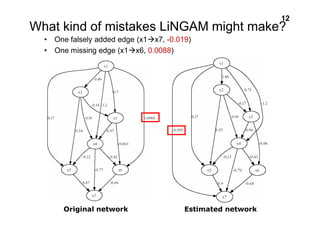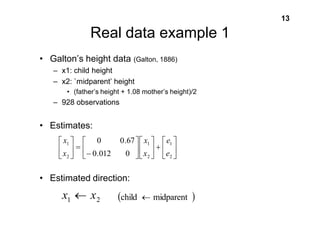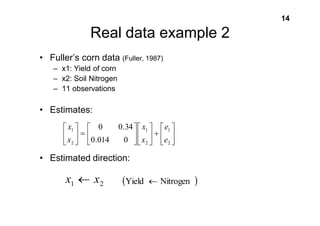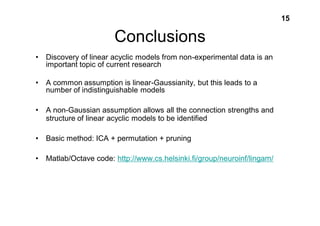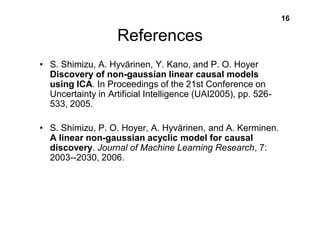1 de 16
Anúncio

### Discovery of Linear Acyclic Models Using Independent Component Analysis

1. Created by S.S. in Jan 2008 Discovery of Linear Acyclic Models Using Independent Component Analysis Shohei Shimizu, Patrik Hoyer, Aapo Hyvarinen and Antti Kerminen LiNGAM homepage: http://www.cs.helsinki.fi/group/neuroinf/lingam/
2. 2 Independent Component Analysis (Hyvarinen et al., 2001) x As m 㬍1 m 㬍n n 㬍1 • A is unknown, cov(s)=I – Typically, A is square (m=n) i s • are assumed to be non-Gaussian and mutually independent • Non-Gaussian and independence are the key assumptions in ICA • Estimable including the rotation (Comon, 1994)
3. 3 Linear acyclic models (Bollen, 1989; Pearl, 2000; Spirtes et al., 2000) • Continuous variables • Directed acyclic graph (DAG) • The value assigned to each variable is a linear combination of those previously assigned, plus a disturbance term ei • Disturbances (errors) are independent and have non-zero variances x Bx e i i ij j e x b x k j k i ( ) ( ) or
4. 4 Our goal • We know – Data X is generated by • We do NOT know x Bx e – Connection strengths: B – Order: k(i) – Disturbances: ei • What we observe is data X only • Goal – Estimate B and k using data X only!
5. 5 Can we recover the original network? Original network ?
6. 6 Can we recover the original network using ICA? Yes! Original network Estimated network
7. 7 Discovery of linear acyclic models from non-experimental data • Existing methods (Bollen,1989; Pearl, 2000; Spirtes et al., 2000) – Gaussian assumption on disturbances ei – Produce many equivalent models • Our LiNGAM approach (Shimizu et al, UAI2005, 2006 JMLR) – Replace Gaussian assumption by non-Gaussian assumption – Can identify the connection strengths and structure Gaussnianity Non-Gaussianity Equivalent models No Equivalent models x1 x2 x3 x1 x2 x3 x1 x2 x3 x1 x2 x3 x1 x2 x3 x1 x2 x3
8. 8 Linear Non-Gaussian Acyclic Models (LiNGAM) • As usual, linear acyclic models, but disturbances are assumed to be non-Gaussian: i ij j e x b x • Examples x Bx e i k j k i ( ) ( ) or i e
9. Outline of LiNGAM algorithm 9 x2 x3 x4 x1 Non-significant edges x2 x3 x4 x1 1. Estimate B by ICA + post-processing 2. Find an order k(i) (DAG) 3. Prune non-significant edges x2 x3 x4 x1
10. 10 Key ideas i x • Observed variables are linear combinations of non-Gaussian independent disturbances x Bx e ( )1 x I B e Ae – The classic case of ICA (Independent Component Analysis) • Permutation indeterminacy in ICA can be solved – Can be shown that the correct permutation is the only one which has no zeros in the diagonal (Shimizu et al., 2005; 2006) • Pruning edges can be done by many existing methods – Imposing sparseness, testing, model fit etc. i e
11. 11 Examples of estimated networks • All the edges correctly identified • All the connection strengths approximately correct Original network Estimated network
12. 12 What kind of mistakes LiNGAM might make? • One falsely added edge (x1x7, -0.019) • One missing edge (x1x6, 0.0088) Original network Estimated network
13. 13 Real data example 1 • Galton’s height data (Galton, 1886) – x1: child height – x2: `midparent’ height • (father’s height + 1.08 mother’s height)/2 – 928 observations • Estimates: x 1 0 0.67 • Estimated direction:
14. e 1 2 x 1 2 2 0.012 0 e x x 1 2 x x child midparent
15. 14 Real data example 2 • Fuller’s corn data (Fuller, 1987) – x1: Yield of corn – x2: Soil Nitrogen – 11 observations • Estimates: x 1 0 0.34 • Estimated direction:
16. e 1 2 x 1 2 2 0.014 0 e x x 1 2 x x Yield Nitrogen
17. 15 Conclusions • Discovery of linear acyclic models from non-experimental data is an important topic of current research • A common assumption is linear-Gaussianity, but this leads to a number of indistinguishable models • A non-Gaussian assumption allows all the connection strengths and structure of linear acyclic models to be identified • Basic method: ICA + permutation + pruning • Matlab/Octave code: http://www.cs.helsinki.fi/group/neuroinf/lingam/
18. 16 References • S. Shimizu, A. Hyvärinen, Y. Kano, and P. O. Hoyer Discovery of non-gaussian linear causal models using ICA. In Proceedings of the 21st Conference on Uncertainty in Artificial Intelligence (UAI2005), pp. 526- 533, 2005. • S. Shimizu, P. O. Hoyer, A. Hyvärinen, and A. Kerminen. A linear non-gaussian acyclic model for causal discovery. Journal of Machine Learning Research, 7: 2003--2030, 2006.
Anúncio